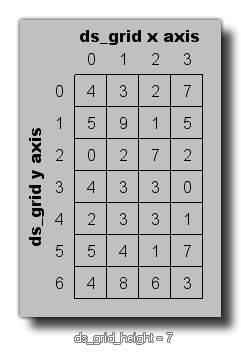## ds_grid_height

### 描述

This function will return the height of the given grid. This value is the number of cells the grid has along the y-axis and is always an integer, as shown in the image below:### 语法：

ds_grid_height(index);

index This index of the grid to find the height of.

Real（实数）

### 例如：

var i, j;
for (i = 0; i < ds_grid_width(grid); i += 1)
{
for (j = 0; j < ds_grid_height(grid); j += 1)
{
if ds_grid_get(grid, i, j) = 1
{
instance_create_Layer(i * 32, j * 32, "Walls", obj_Wall);
}
}
}